33 Electric Fields and Potential An electric field

• Slides: 84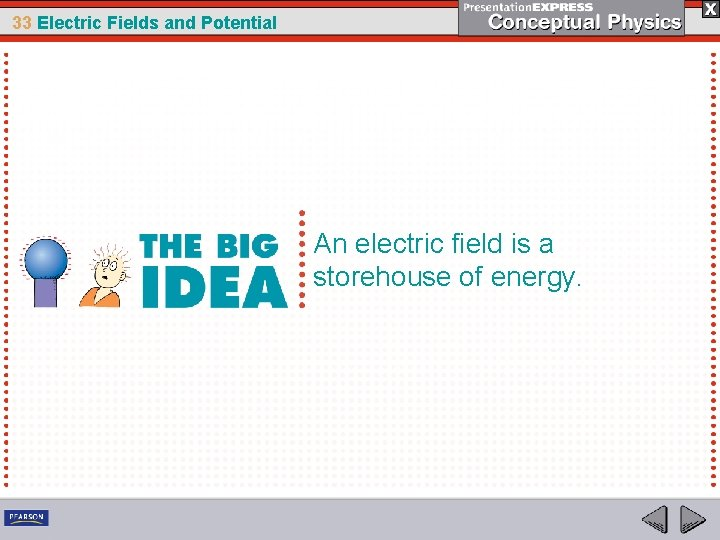33 Electric Fields and Potential An electric field is a storehouse of energy.33 Electric Fields and Potential The space around a concentration of electric charge is different from how it would be if the charge were not there. If you walk by the charged dome of an electrostatic machine—a Van de Graaff generator, for example— you can sense the charge. Hair on your body stands out—just a tiny bit if you’re more than a meter away, and more if you’re closer. The space is said to contain a force field.33 Electric Fields and Potential 33. 1 Electric Fields The magnitude (strength) of an electric field can be measured by its effect on charges located in the field. The direction of an electric field at any point, by convention, is the direction of the electrical force on a small positive test charge placed at that point.33 Electric Fields and Potential 33. 1 Electric Fields If you throw a ball upward, it follows a curved path due to interaction between the centers of gravity of the ball and Earth. The centers of gravity are far apart, so this is “action at a distance. ” The concept of a force field explains how Earth can exert a force on things without touching them. The ball is in contact with the field all the time.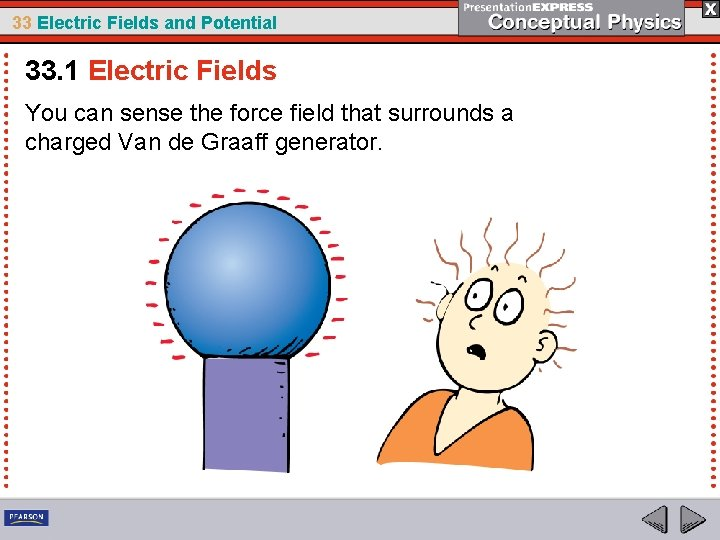33 Electric Fields and Potential 33. 1 Electric Fields You can sense the force field that surrounds a charged Van de Graaff generator.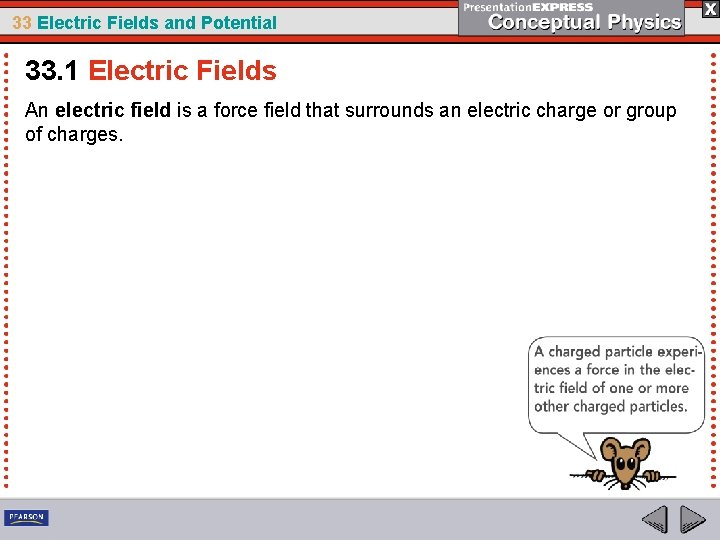33 Electric Fields and Potential 33. 1 Electric Fields An electric field is a force field that surrounds an electric charge or group of charges.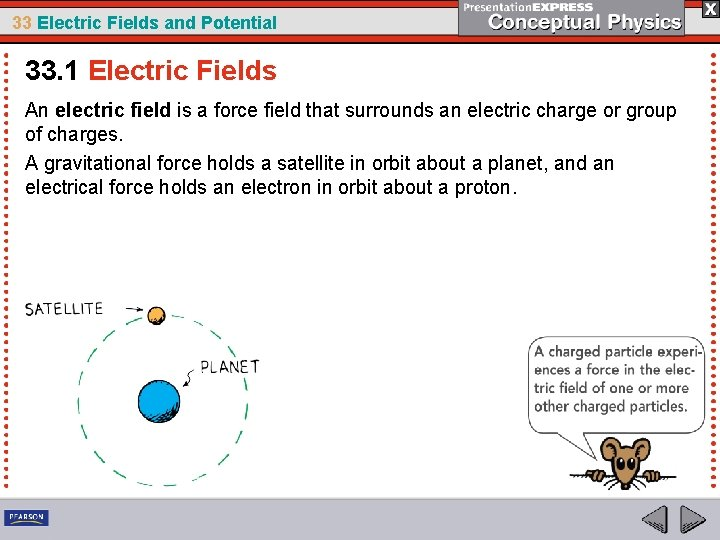33 Electric Fields and Potential 33. 1 Electric Fields An electric field is a force field that surrounds an electric charge or group of charges. A gravitational force holds a satellite in orbit about a planet, and an electrical force holds an electron in orbit about a proton.33 Electric Fields and Potential 33. 1 Electric Fields An electric field is a force field that surrounds an electric charge or group of charges. A gravitational force holds a satellite in orbit about a planet, and an electrical force holds an electron in orbit about a proton. The force that one electric charge exerts on another is the interaction between one charge and the electric field of the other.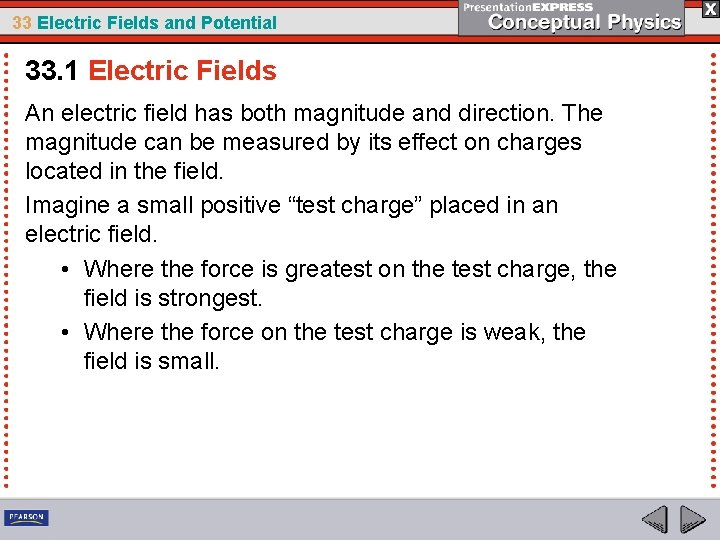33 Electric Fields and Potential 33. 1 Electric Fields An electric field has both magnitude and direction. The magnitude can be measured by its effect on charges located in the field. Imagine a small positive “test charge” placed in an electric field. • Where the force is greatest on the test charge, the field is strongest. • Where the force on the test charge is weak, the field is small.33 Electric Fields and Potential 33. 1 Electric Fields The direction of an electric field at any point, by convention, is the direction of the electrical force on a small positive test charge. • If the charge that sets up the field is positive, the field points away from that charge. • If the charge that sets up the field is negative, the field points toward that charge.33 Electric Fields and Potential 33. 1 Electric Fields How are the magnitude and direction of an electric field determined?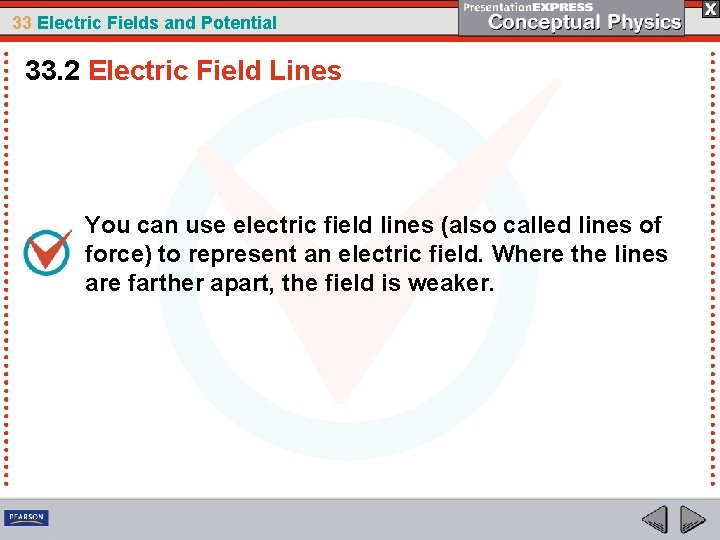33 Electric Fields and Potential 33. 2 Electric Field Lines You can use electric field lines (also called lines of force) to represent an electric field. Where the lines are farther apart, the field is weaker.33 Electric Fields and Potential 33. 2 Electric Field Lines Since an electric field has both magnitude and direction, it is a vector quantity and can be represented by vectors. • A negatively charged particle is surrounded by vectors that point toward the particle. • For a positively charged particle, the vectors point away. • Magnitude of the field is indicated by the vector length. The electric field is greater where the vectors are longer.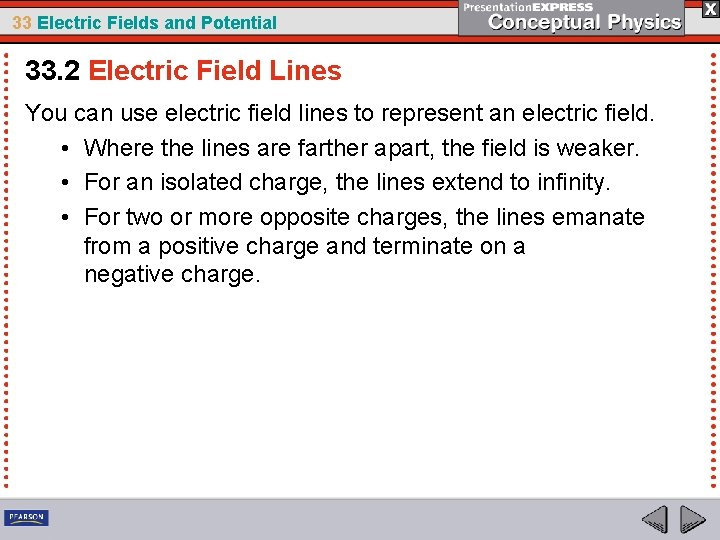33 Electric Fields and Potential 33. 2 Electric Field Lines You can use electric field lines to represent an electric field. • Where the lines are farther apart, the field is weaker. • For an isolated charge, the lines extend to infinity. • For two or more opposite charges, the lines emanate from a positive charge and terminate on a negative charge.33 Electric Fields and Potential 33. 2 Electric Field Lines a. In a vector representation of an electric field, the length of the vectors indicates the magnitude of the field.33 Electric Fields and Potential 33. 2 Electric Field Lines a. In a vector representation of an electric field, the length of the vectors indicates the magnitude of the field. b. In a lines of force representation, the distance between field lines indicates magnitudes.33 Electric Fields and Potential 33. 2 Electric Field Lines a. The field lines around a single positive charge extend to infinity.33 Electric Fields and Potential 33. 2 Electric Field Lines a. b. The field lines around a single positive charge extend to infinity. For a pair of equal but opposite charges, the field lines emanate from the positive charge and terminate on the negative charge.33 Electric Fields and Potential 33. 2 Electric Field Lines a. b. c. The field lines around a single positive charge extend to infinity. For a pair of equal but opposite charges, the field lines emanate from the positive charge and terminate on the negative charge. Field lines are evenly spaced between two oppositely charged capacitor plates.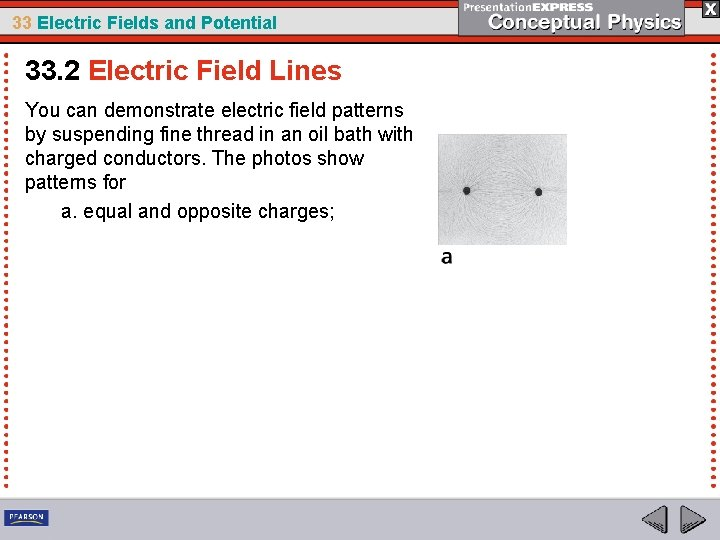33 Electric Fields and Potential 33. 2 Electric Field Lines You can demonstrate electric field patterns by suspending fine thread in an oil bath with charged conductors. The photos show patterns for a. equal and opposite charges;33 Electric Fields and Potential 33. 2 Electric Field Lines You can demonstrate electric field patterns by suspending fine thread in an oil bath with charged conductors. The photos show patterns for a. equal and opposite charges; b. equal like charges;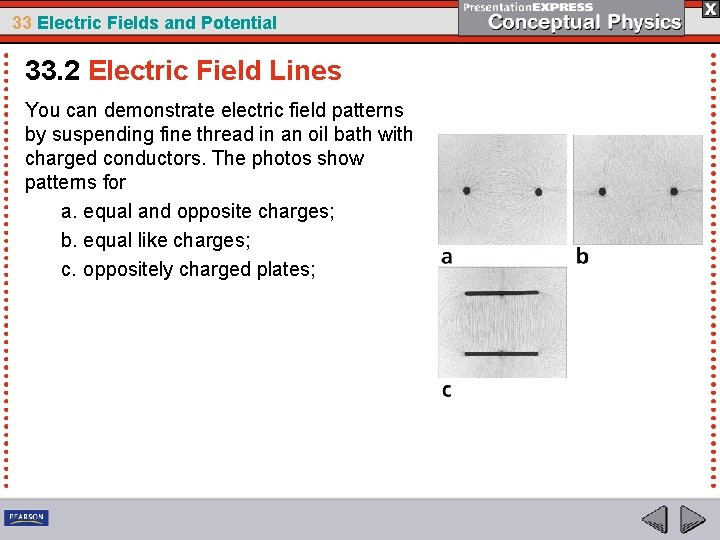33 Electric Fields and Potential 33. 2 Electric Field Lines You can demonstrate electric field patterns by suspending fine thread in an oil bath with charged conductors. The photos show patterns for a. equal and opposite charges; b. equal like charges; c. oppositely charged plates;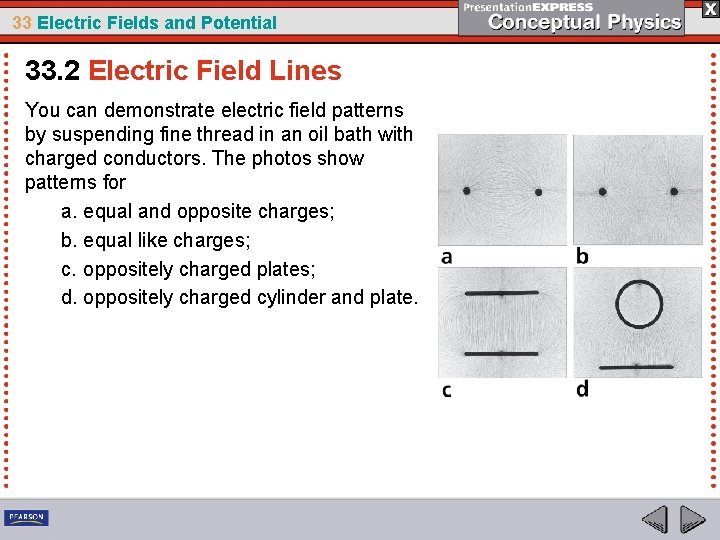33 Electric Fields and Potential 33. 2 Electric Field Lines You can demonstrate electric field patterns by suspending fine thread in an oil bath with charged conductors. The photos show patterns for a. equal and opposite charges; b. equal like charges; c. oppositely charged plates; d. oppositely charged cylinder and plate.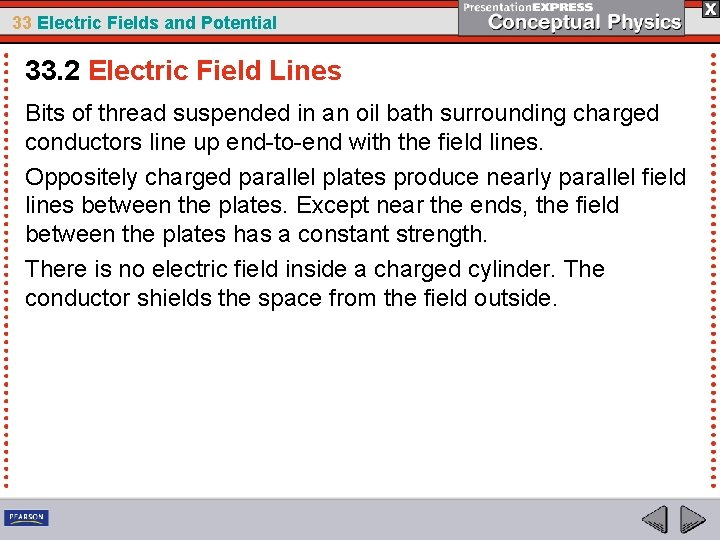33 Electric Fields and Potential 33. 2 Electric Field Lines Bits of thread suspended in an oil bath surrounding charged conductors line up end to end with the field lines. Oppositely charged parallel plates produce nearly parallel field lines between the plates. Except near the ends, the field between the plates has a constant strength. There is no electric field inside a charged cylinder. The conductor shields the space from the field outside.33 Electric Fields and Potential 33. 2 Electric Field Lines think! A beam of electrons is produced at one end of a glass tube and lights up a phosphor screen at the other end. If the beam passes through the electric field of a pair of oppositely charged plates, it is deflected upward as shown. If the charges on the plates are reversed, in what direction will the beam deflect?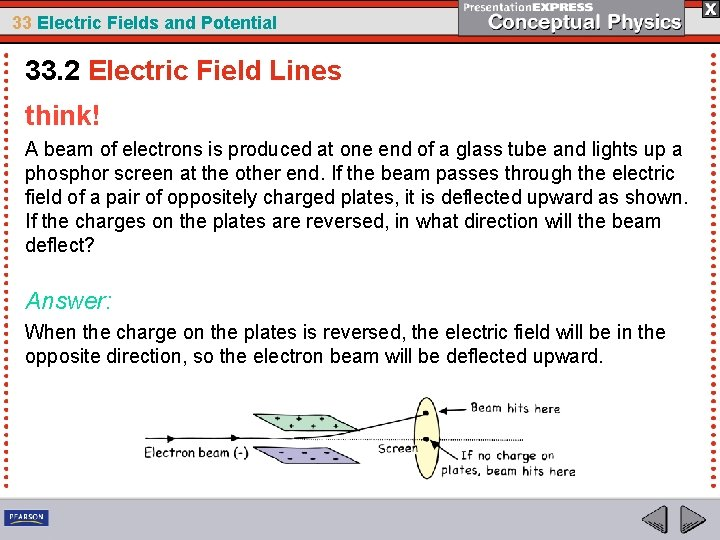33 Electric Fields and Potential 33. 2 Electric Field Lines think! A beam of electrons is produced at one end of a glass tube and lights up a phosphor screen at the other end. If the beam passes through the electric field of a pair of oppositely charged plates, it is deflected upward as shown. If the charges on the plates are reversed, in what direction will the beam deflect? Answer: When the charge on the plates is reversed, the electric field will be in the opposite direction, so the electron beam will be deflected upward.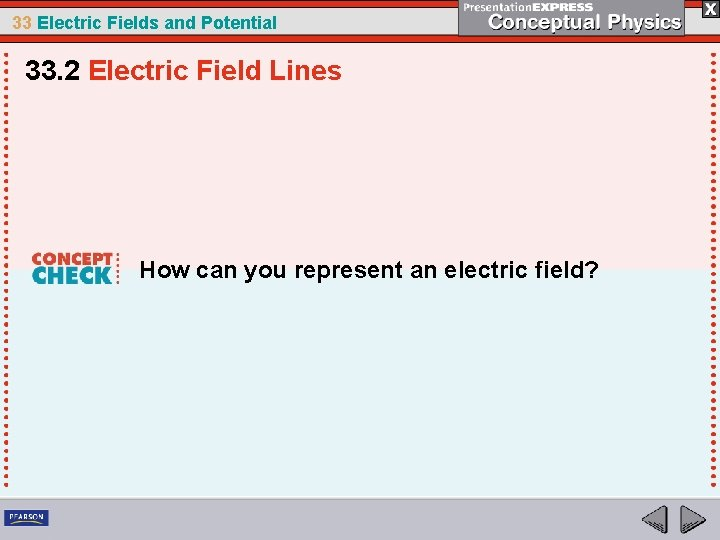33 Electric Fields and Potential 33. 2 Electric Field Lines How can you represent an electric field?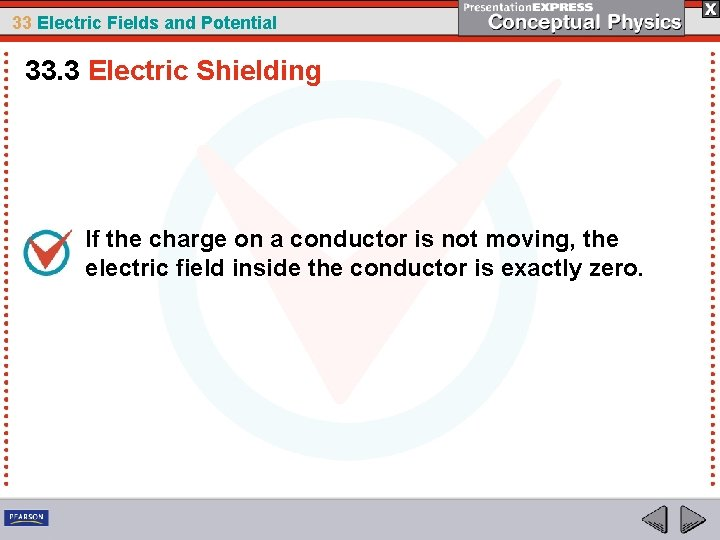33 Electric Fields and Potential 33. 3 Electric Shielding If the charge on a conductor is not moving, the electric field inside the conductor is exactly zero.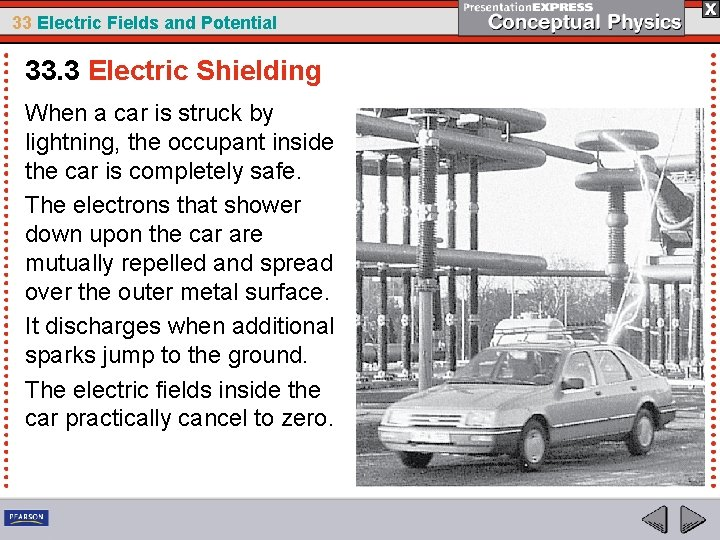33 Electric Fields and Potential 33. 3 Electric Shielding When a car is struck by lightning, the occupant inside the car is completely safe. The electrons that shower down upon the car are mutually repelled and spread over the outer metal surface. It discharges when additional sparks jump to the ground. The electric fields inside the car practically cancel to zero.33 Electric Fields and Potential 33. 3 Electric Shielding Charged Conductors The absence of electric field within a conductor holding static charge is not an inability of an electric field to penetrate metals. Free electrons within the conductor can “settle down” and stop moving only when the electric field is zero. The charges arrange to ensure a zero field with the material.33 Electric Fields and Potential 33. 3 Electric Shielding Consider a charged metal sphere. Because of repulsion, electrons spread as far apart as possible, uniformly over the surface. A positive test charge located exactly in the middle of the sphere would feel no force. The net force on a test charge would be zero. The electric field is also zero. Complete cancellation will occur anywhere inside the sphere.33 Electric Fields and Potential 33. 3 Electric Shielding If the conductor is not spherical, the charge distribution will not be uniform but the electric field inside the conductor is zero. If there were an electric field inside a conductor, then free electrons inside the conductor would be set in motion. They would move to establish equilibrium, that is, all the electrons produce a zero field inside the conductor.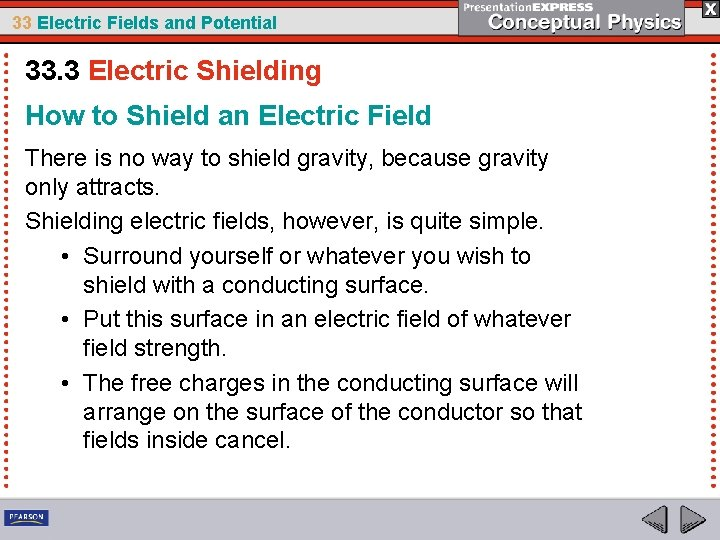33 Electric Fields and Potential 33. 3 Electric Shielding How to Shield an Electric Field There is no way to shield gravity, because gravity only attracts. Shielding electric fields, however, is quite simple. • Surround yourself or whatever you wish to shield with a conducting surface. • Put this surface in an electric field of whatever field strength. • The free charges in the conducting surface will arrange on the surface of the conductor so that fields inside cancel.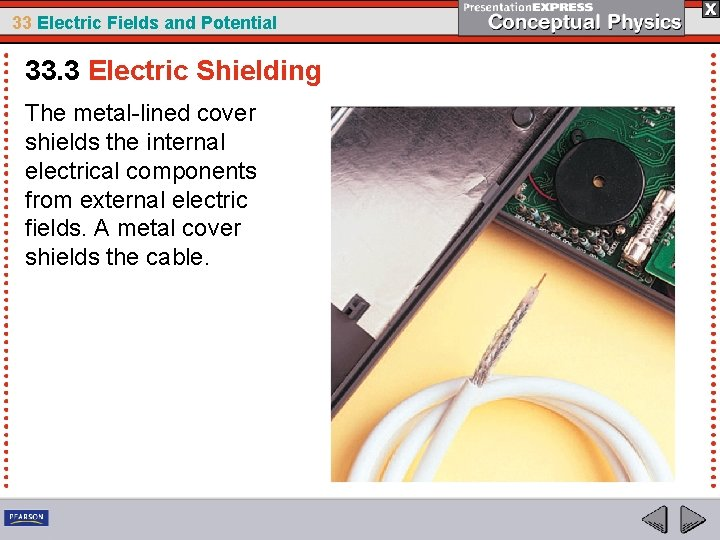33 Electric Fields and Potential 33. 3 Electric Shielding The metal lined cover shields the internal electrical components from external electric fields. A metal cover shields the cable.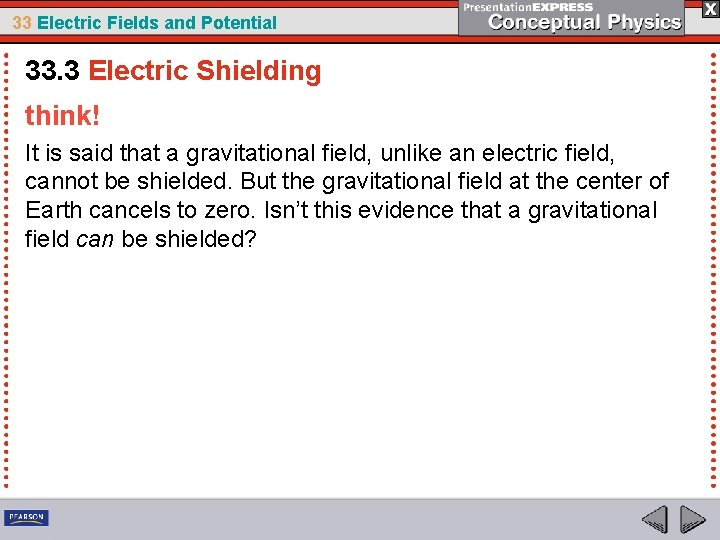33 Electric Fields and Potential 33. 3 Electric Shielding think! It is said that a gravitational field, unlike an electric field, cannot be shielded. But the gravitational field at the center of Earth cancels to zero. Isn’t this evidence that a gravitational field can be shielded?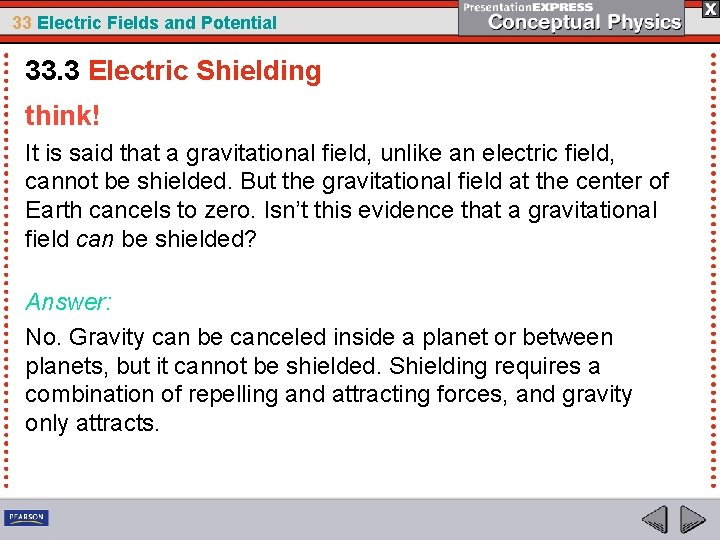33 Electric Fields and Potential 33. 3 Electric Shielding think! It is said that a gravitational field, unlike an electric field, cannot be shielded. But the gravitational field at the center of Earth cancels to zero. Isn’t this evidence that a gravitational field can be shielded? Answer: No. Gravity can be canceled inside a planet or between planets, but it cannot be shielded. Shielding requires a combination of repelling and attracting forces, and gravity only attracts.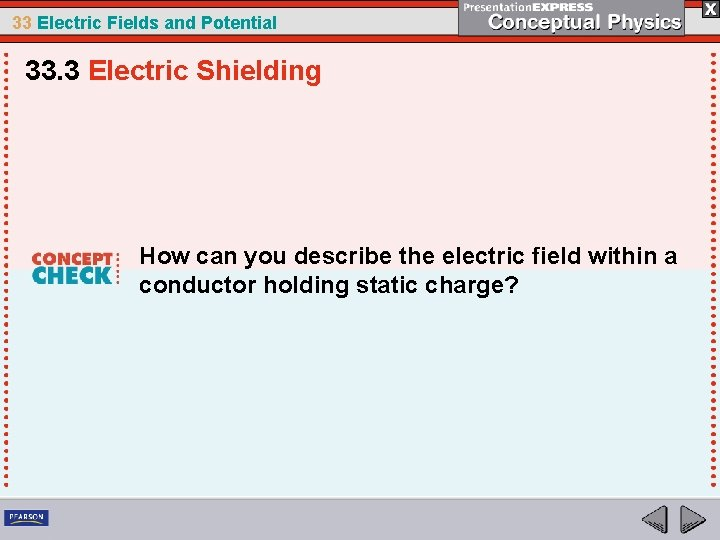33 Electric Fields and Potential 33. 3 Electric Shielding How can you describe the electric field within a conductor holding static charge?33 Electric Fields and Potential 33. 4 Electrical Potential Energy The electrical potential energy of a charged particle is increased when work is done to push it against the electric field of something else that is charged.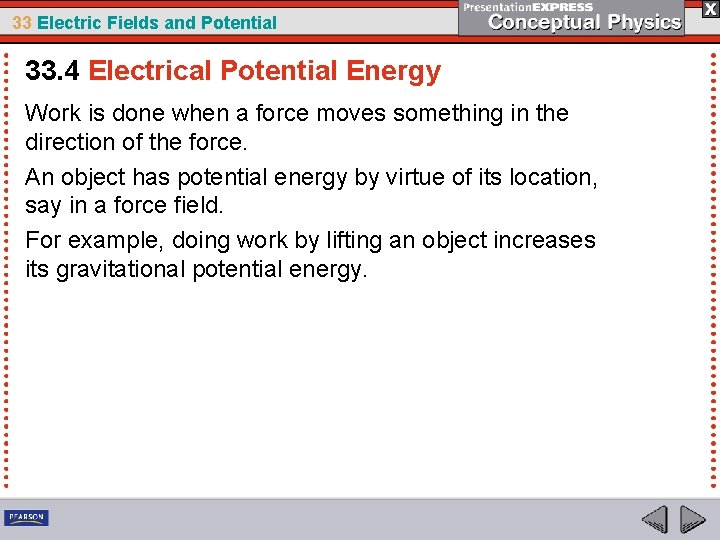33 Electric Fields and Potential 33. 4 Electrical Potential Energy Work is done when a force moves something in the direction of the force. An object has potential energy by virtue of its location, say in a force field. For example, doing work by lifting an object increases its gravitational potential energy.33 Electric Fields and Potential 33. 4 Electrical Potential Energy a. In an elevated position, the ram has gravitational potential energy. When released, this energy is transferred to the pile below.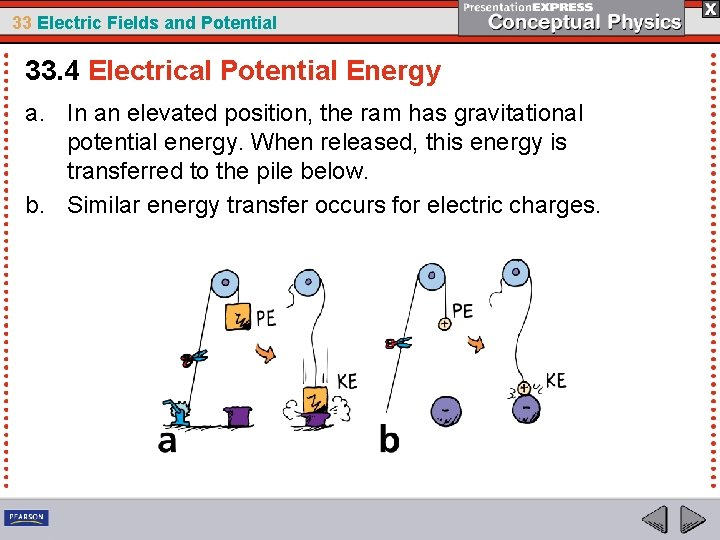33 Electric Fields and Potential 33. 4 Electrical Potential Energy a. In an elevated position, the ram has gravitational potential energy. When released, this energy is transferred to the pile below. b. Similar energy transfer occurs for electric charges.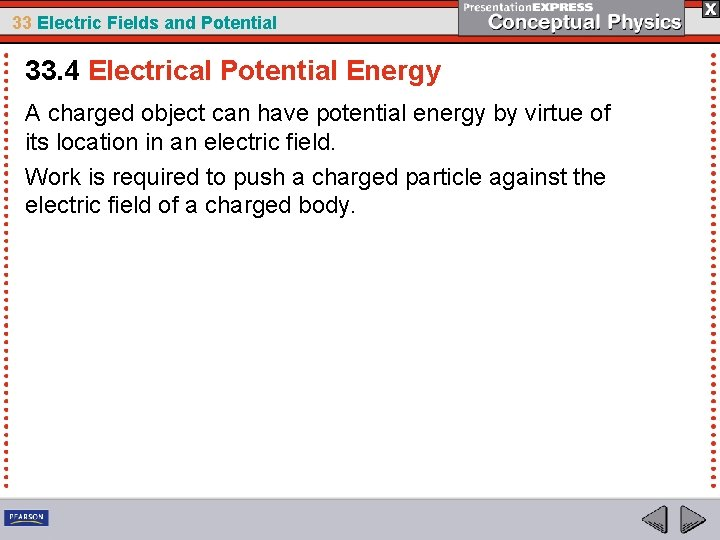33 Electric Fields and Potential 33. 4 Electrical Potential Energy A charged object can have potential energy by virtue of its location in an electric field. Work is required to push a charged particle against the electric field of a charged body.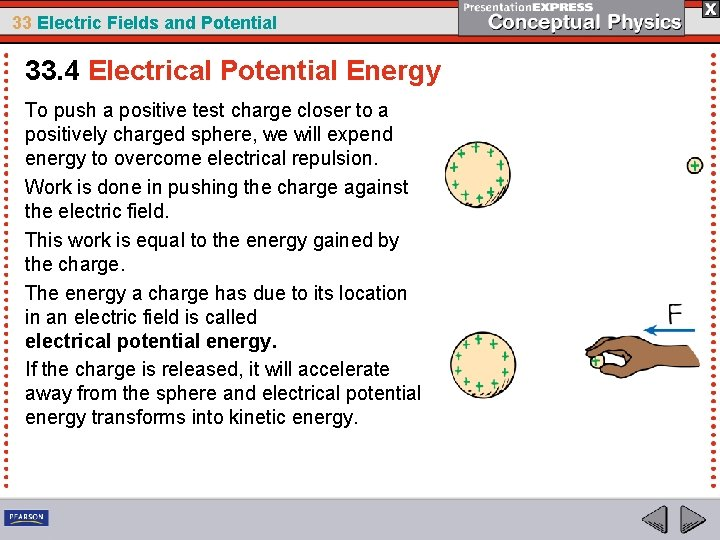33 Electric Fields and Potential 33. 4 Electrical Potential Energy To push a positive test charge closer to a positively charged sphere, we will expend energy to overcome electrical repulsion. Work is done in pushing the charge against the electric field. This work is equal to the energy gained by the charge. The energy a charge has due to its location in an electric field is called electrical potential energy. If the charge is released, it will accelerate away from the sphere and electrical potential energy transforms into kinetic energy.33 Electric Fields and Potential 33. 4 Electrical Potential Energy How can you increase the electrical potential energy of a charged particle?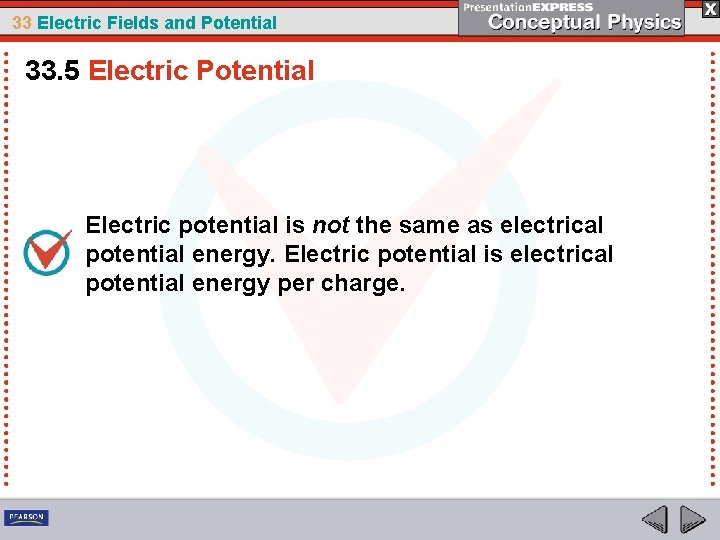33 Electric Fields and Potential 33. 5 Electric Potential Electric potential is not the same as electrical potential energy. Electric potential is electrical potential energy per charge.33 Electric Fields and Potential 33. 5 Electric Potential If we push a single charge against an electric field, we do a certain amount of work. If we push two charges against the same field, we do twice as much work. Two charges in the same location in an electric field will have twice the electrical potential energy as one; ten charges will have ten times the potential energy. It is convenient when working with electricity to consider the electrical potential energy per charge.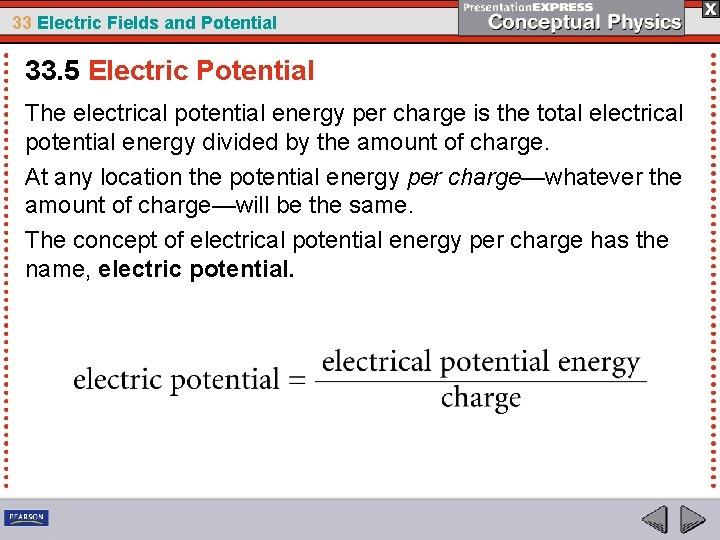33 Electric Fields and Potential 33. 5 Electric Potential The electrical potential energy per charge is the total electrical potential energy divided by the amount of charge. At any location the potential energy per charge—whatever the amount of charge—will be the same. The concept of electrical potential energy per charge has the name, electric potential.33 Electric Fields and Potential 33. 5 Electric Potential An object of greater charge has more electrical potential energy in the field of the charged dome than an object of less charge, but the electric potential of any charge at the same location is the same.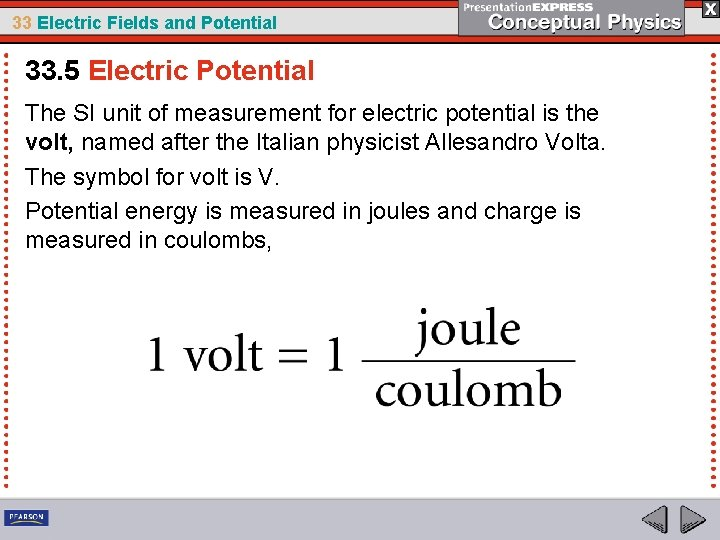33 Electric Fields and Potential 33. 5 Electric Potential The SI unit of measurement for electric potential is the volt, named after the Italian physicist Allesandro Volta. The symbol for volt is V. Potential energy is measured in joules and charge is measured in coulombs,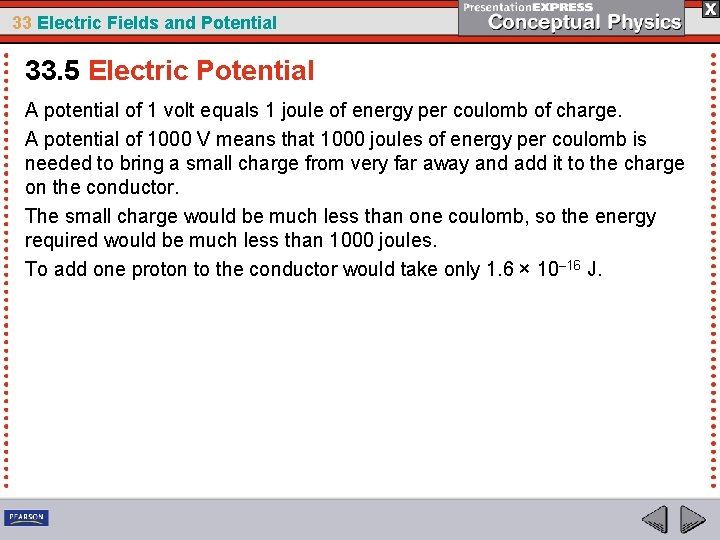33 Electric Fields and Potential 33. 5 Electric Potential A potential of 1 volt equals 1 joule of energy per coulomb of charge. A potential of 1000 V means that 1000 joules of energy per coulomb is needed to bring a small charge from very far away and add it to the charge on the conductor. The small charge would be much less than one coulomb, so the energy required would be much less than 1000 joules. To add one proton to the conductor would take only 1. 6 × 10– 16 J.33 Electric Fields and Potential 33. 5 Electric Potential Since electric potential is measured in volts, it is commonly called voltage. Once the location of zero voltage has been specified, a definite value for it can be assigned to a location whether or not a charge exists at that location. We can speak about the voltages at different locations in an electric field whether or not any charges occupy those locations.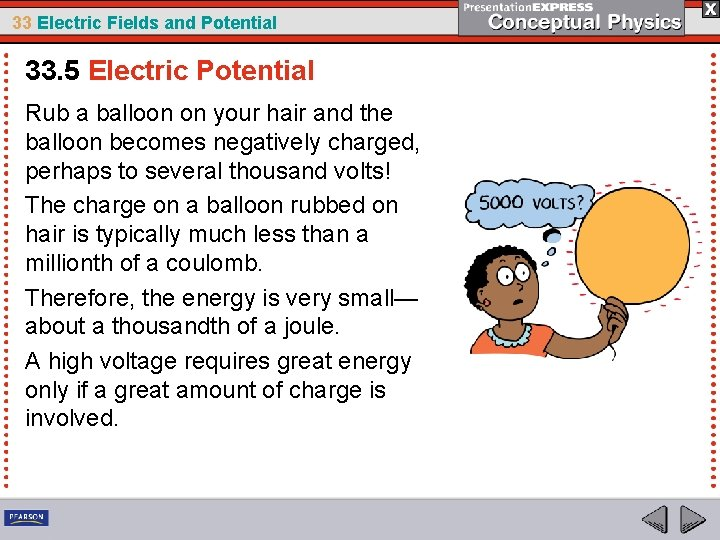33 Electric Fields and Potential 33. 5 Electric Potential Rub a balloon on your hair and the balloon becomes negatively charged, perhaps to several thousand volts! The charge on a balloon rubbed on hair is typically much less than a millionth of a coulomb. Therefore, the energy is very small— about a thousandth of a joule. A high voltage requires great energy only if a great amount of charge is involved.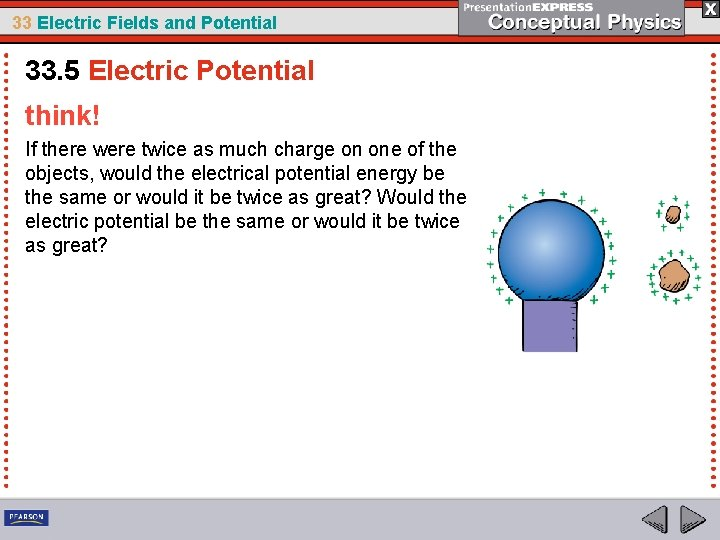33 Electric Fields and Potential 33. 5 Electric Potential think! If there were twice as much charge on one of the objects, would the electrical potential energy be the same or would it be twice as great? Would the electric potential be the same or would it be twice as great?33 Electric Fields and Potential 33. 5 Electric Potential think! If there were twice as much charge on one of the objects, would the electrical potential energy be the same or would it be twice as great? Would the electric potential be the same or would it be twice as great? Answer: Twice as much charge would cause the object to have twice as much electrical potential energy, because it would have taken twice as much work to bring the object to that location. The electric potential would be the same, because the electric potential is total electrical potential energy divided by total charge.33 Electric Fields and Potential 33. 5 Electric Potential What is the difference between electric potential and electrical potential energy?33 Electric Fields and Potential 33. 6 Electrical Energy Storage The energy stored in a capacitor comes from the work done to charge it.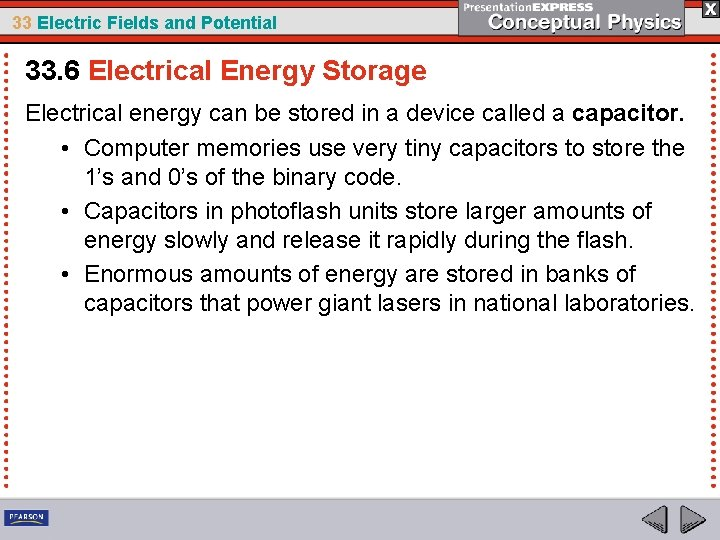33 Electric Fields and Potential 33. 6 Electrical Energy Storage Electrical energy can be stored in a device called a capacitor. • Computer memories use very tiny capacitors to store the 1’s and 0’s of the binary code. • Capacitors in photoflash units store larger amounts of energy slowly and release it rapidly during the flash. • Enormous amounts of energy are stored in banks of capacitors that power giant lasers in national laboratories.33 Electric Fields and Potential 33. 6 Electrical Energy Storage The simplest capacitor is a pair of conducting plates separated by a small distance, but not touching each other. • Charge is transferred from one plate to the other. • The capacitor plates then have equal and opposite charges. • The charging process is complete when the potential difference between the plates equals the potential difference between the battery terminals—the battery voltage. • The greater the battery voltage and the larger and closer the plates, the greater the charge that is stored.33 Electric Fields and Potential 33. 6 Electrical Energy Storage In practice, the plates may be thin metallic foils separated by a thin sheet of paper. This “paper sandwich” is then rolled up to save space and may be inserted into a cylinder.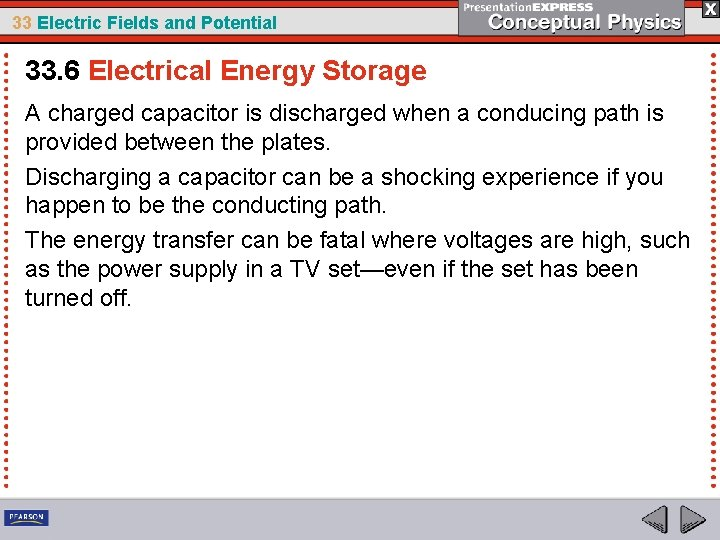33 Electric Fields and Potential 33. 6 Electrical Energy Storage A charged capacitor is discharged when a conducing path is provided between the plates. Discharging a capacitor can be a shocking experience if you happen to be the conducting path. The energy transfer can be fatal where voltages are high, such as the power supply in a TV set—even if the set has been turned off.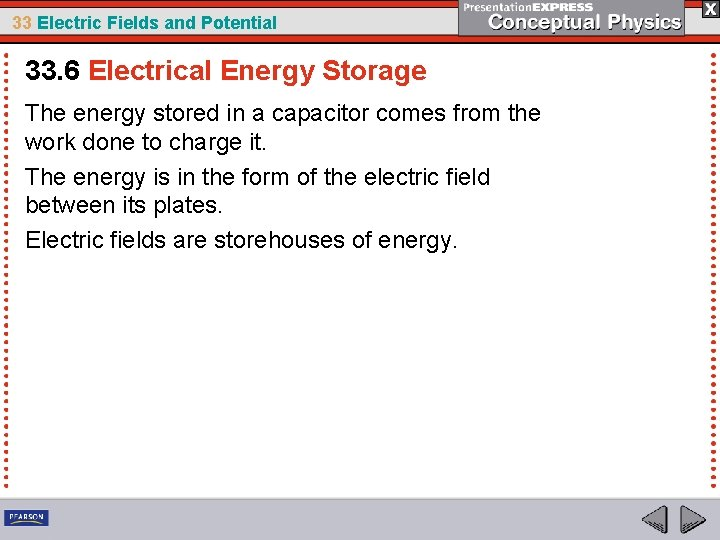33 Electric Fields and Potential 33. 6 Electrical Energy Storage The energy stored in a capacitor comes from the work done to charge it. The energy is in the form of the electric field between its plates. Electric fields are storehouses of energy.33 Electric Fields and Potential 33. 6 Electrical Energy Storage Where does the energy stored in a capacitor come from?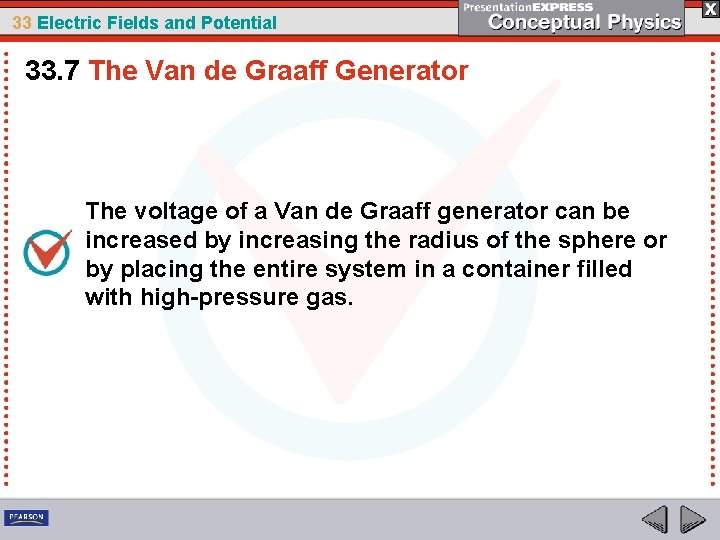33 Electric Fields and Potential 33. 7 The Van de Graaff Generator The voltage of a Van de Graaff generator can be increased by increasing the radius of the sphere or by placing the entire system in a container filled with high-pressure gas.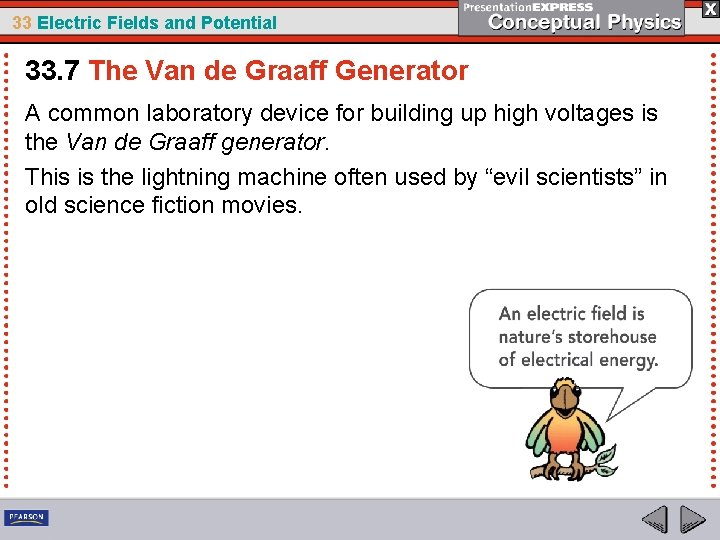33 Electric Fields and Potential 33. 7 The Van de Graaff Generator A common laboratory device for building up high voltages is the Van de Graaff generator. This is the lightning machine often used by “evil scientists” in old science fiction movies.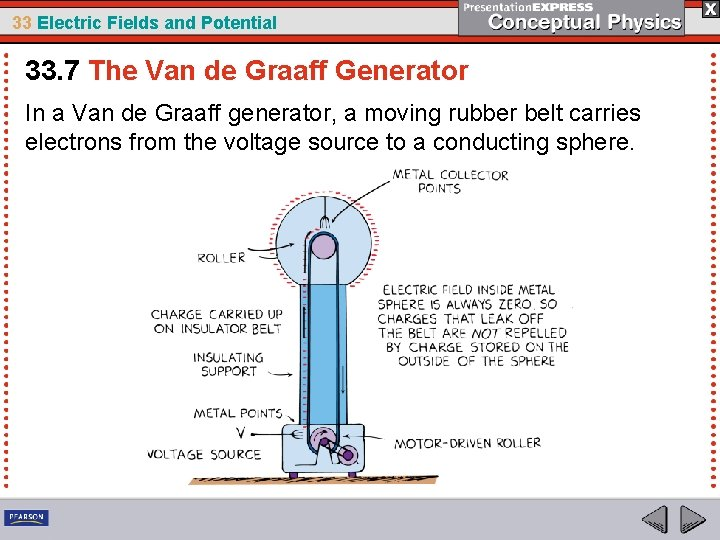33 Electric Fields and Potential 33. 7 The Van de Graaff Generator In a Van de Graaff generator, a moving rubber belt carries electrons from the voltage source to a conducting sphere.33 Electric Fields and Potential 33. 7 The Van de Graaff Generator A large hollow metal sphere is supported by a cylindrical insulating stand. A rubber belt inside the support stand moves past metal needles that are maintained at a high electric potential. A continuous supply of electrons is deposited on the belt through electric discharge by the points of the needles. The electrons are carried up into the hollow metal sphere.33 Electric Fields and Potential 33. 7 The Van de Graaff Generator The electrons leak onto metal points attached to the inner surface of the sphere. Because of mutual repulsion, the electrons move to the outer surface of the conducting sphere. This leaves the inside surface uncharged and able to receive more electrons. The process is continuous, and the charge builds up to a very high electric potential—on the order of millions of volts.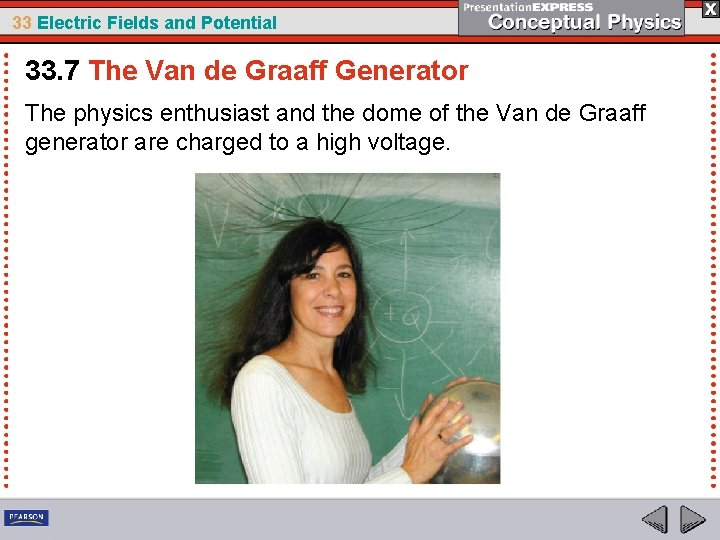33 Electric Fields and Potential 33. 7 The Van de Graaff Generator The physics enthusiast and the dome of the Van de Graaff generator are charged to a high voltage.33 Electric Fields and Potential 33. 7 The Van de Graaff Generator A sphere with a radius of 1 m can be raised to a potential of 3 million volts before electric discharge occurs through the air. The voltage of a Van de Graaff generator can be increased by increasing the radius of the sphere or by placing the entire system in a container filled with high pressuregas. Van de Graaff generators in pressurized gas can produce voltages as high as 20 million volts. These devices accelerate charged particles used as projectiles for penetrating the nuclei of atoms.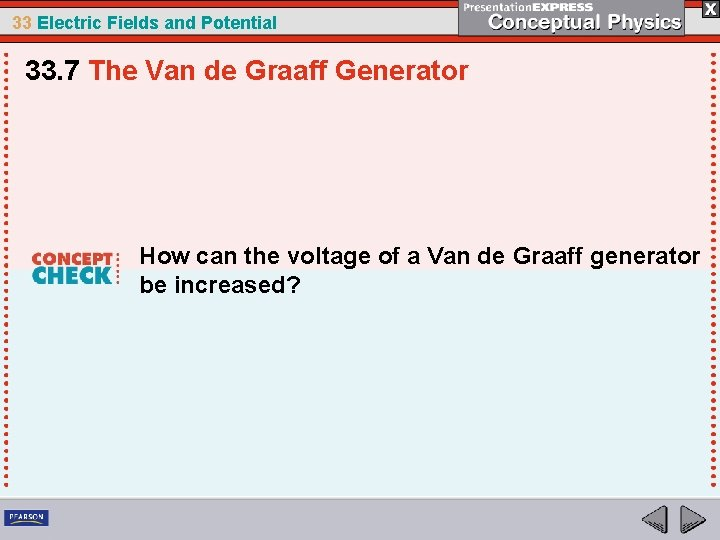33 Electric Fields and Potential 33. 7 The Van de Graaff Generator How can the voltage of a Van de Graaff generator be increased?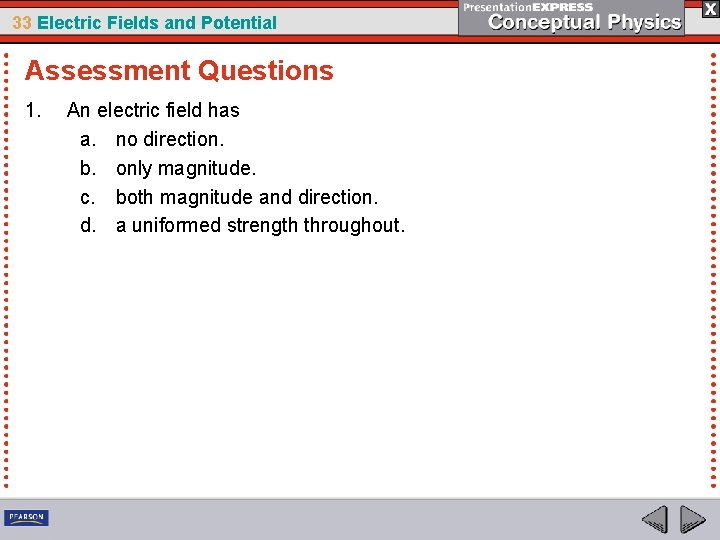33 Electric Fields and Potential Assessment Questions 1. An electric field has a. no direction. b. only magnitude. c. both magnitude and direction. d. a uniformed strength throughout.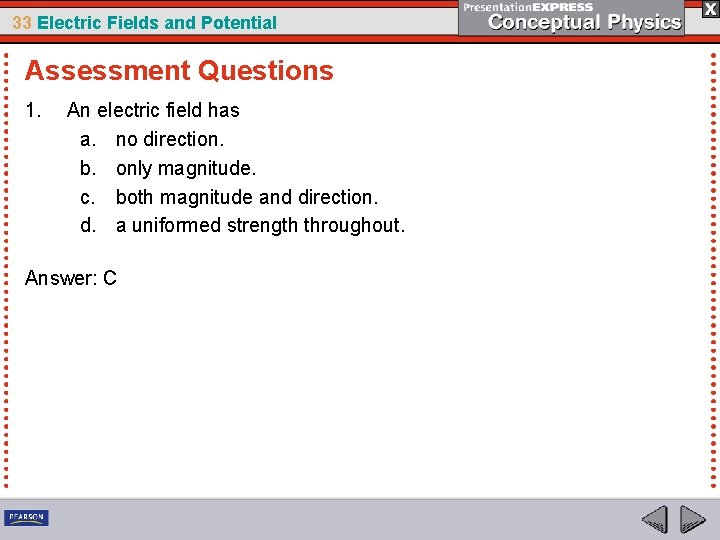33 Electric Fields and Potential Assessment Questions 1. An electric field has a. no direction. b. only magnitude. c. both magnitude and direction. d. a uniformed strength throughout. Answer: C33 Electric Fields and Potential Assessment Questions 2. In the electric field surrounding a group of charged particles, field strength is greater where field lines are a. thickest. b. longest. c. farthest apart. d. closest.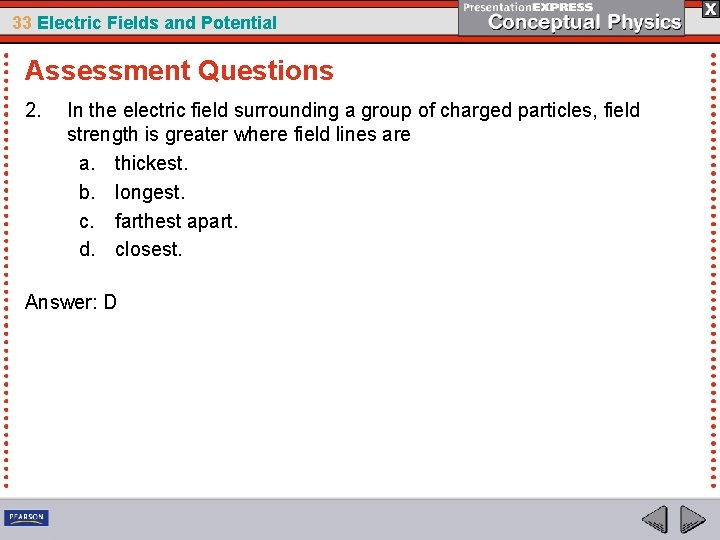33 Electric Fields and Potential Assessment Questions 2. In the electric field surrounding a group of charged particles, field strength is greater where field lines are a. thickest. b. longest. c. farthest apart. d. closest. Answer: D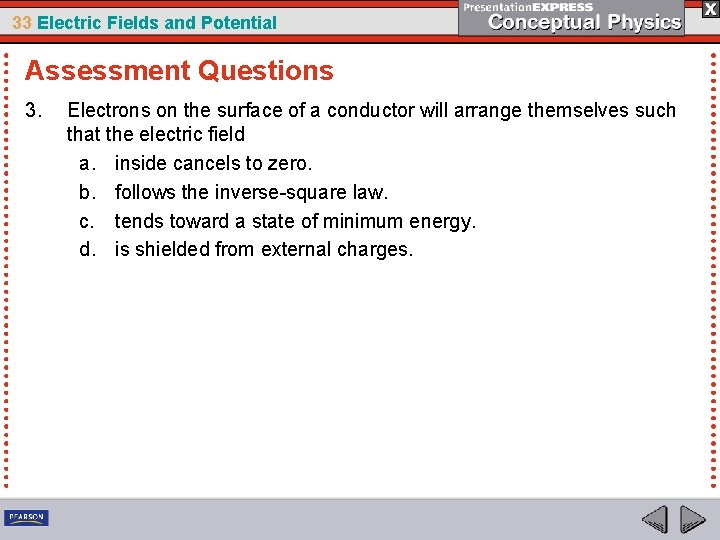33 Electric Fields and Potential Assessment Questions 3. Electrons on the surface of a conductor will arrange themselves such that the electric field a. inside cancels to zero. b. follows the inverse square law. c. tends toward a state of minimum energy. d. is shielded from external charges.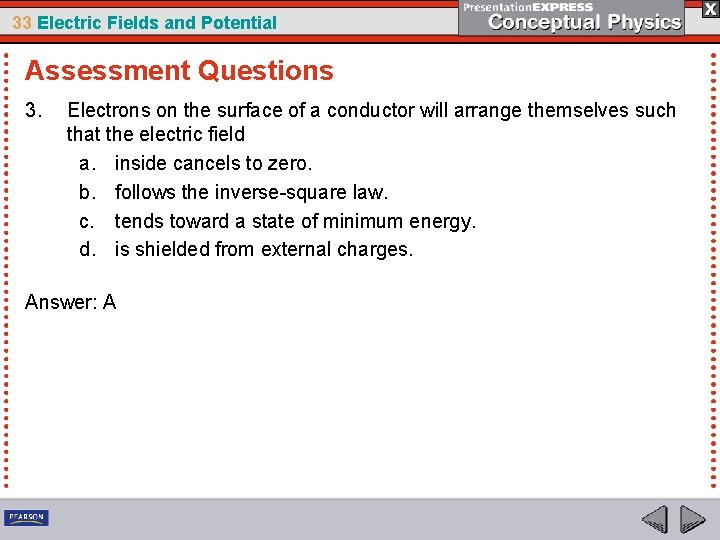33 Electric Fields and Potential Assessment Questions 3. Electrons on the surface of a conductor will arrange themselves such that the electric field a. inside cancels to zero. b. follows the inverse square law. c. tends toward a state of minimum energy. d. is shielded from external charges. Answer: A33 Electric Fields and Potential Assessment Questions 4. The potential energy of a compressed spring and the potential energy of a charged object both depend a. only on the work done on them. b. only on their locations in their respective fields. c. on their locations in their respective fields and on the work done on them. d. on their kinetic energies exceeding their potential energies.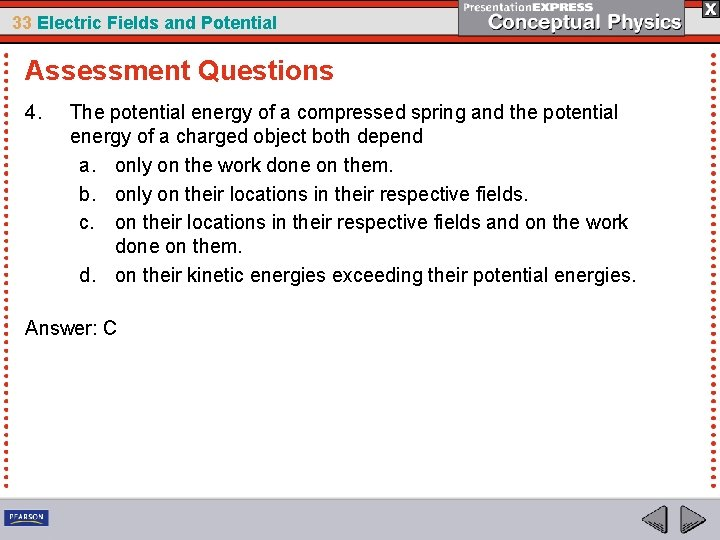33 Electric Fields and Potential Assessment Questions 4. The potential energy of a compressed spring and the potential energy of a charged object both depend a. only on the work done on them. b. only on their locations in their respective fields. c. on their locations in their respective fields and on the work done on them. d. on their kinetic energies exceeding their potential energies. Answer: C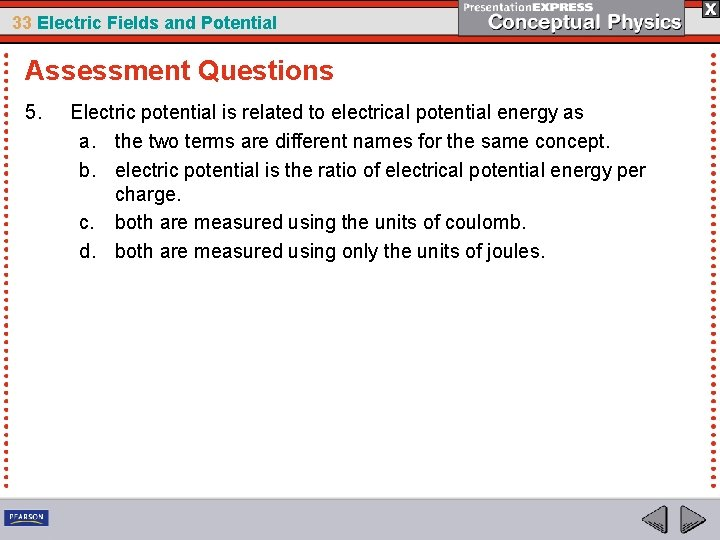33 Electric Fields and Potential Assessment Questions 5. Electric potential is related to electrical potential energy as a. the two terms are different names for the same concept. b. electric potential is the ratio of electrical potential energy per charge. c. both are measured using the units of coulomb. d. both are measured using only the units of joules.33 Electric Fields and Potential Assessment Questions 5. Electric potential is related to electrical potential energy as a. the two terms are different names for the same concept. b. electric potential is the ratio of electrical potential energy per charge. c. both are measured using the units of coulomb. d. both are measured using only the units of joules. Answer: B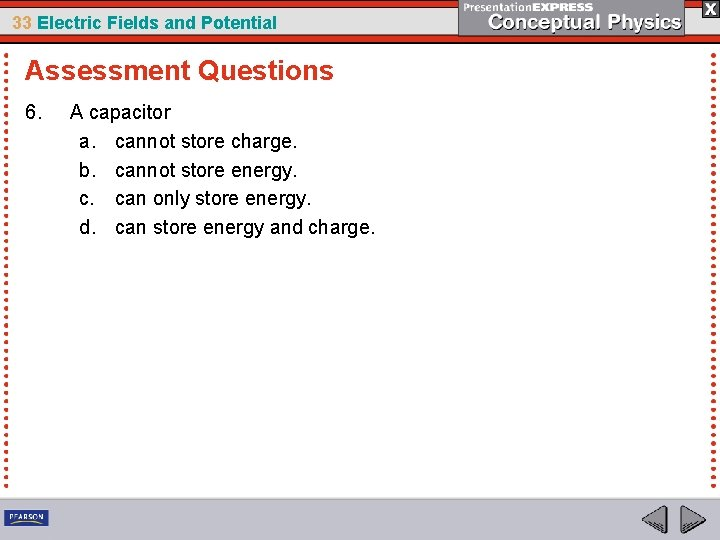33 Electric Fields and Potential Assessment Questions 6. A capacitor a. cannot store charge. b. cannot store energy. c. can only store energy. d. can store energy and charge.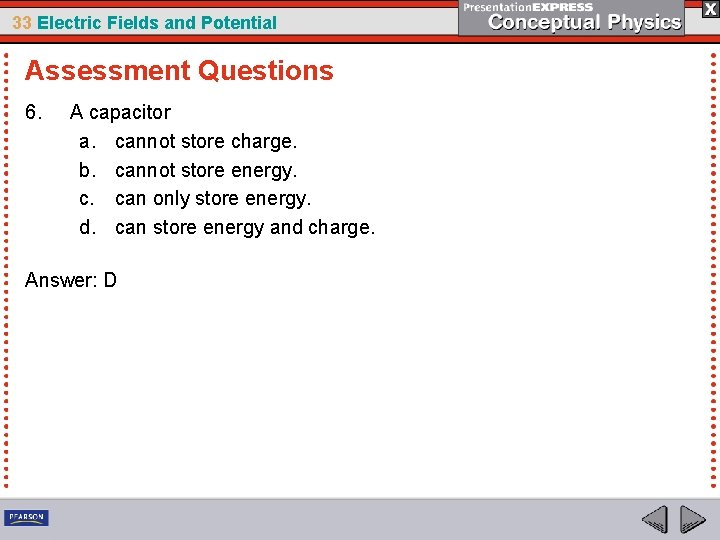33 Electric Fields and Potential Assessment Questions 6. A capacitor a. cannot store charge. b. cannot store energy. c. can only store energy. d. can store energy and charge. Answer: D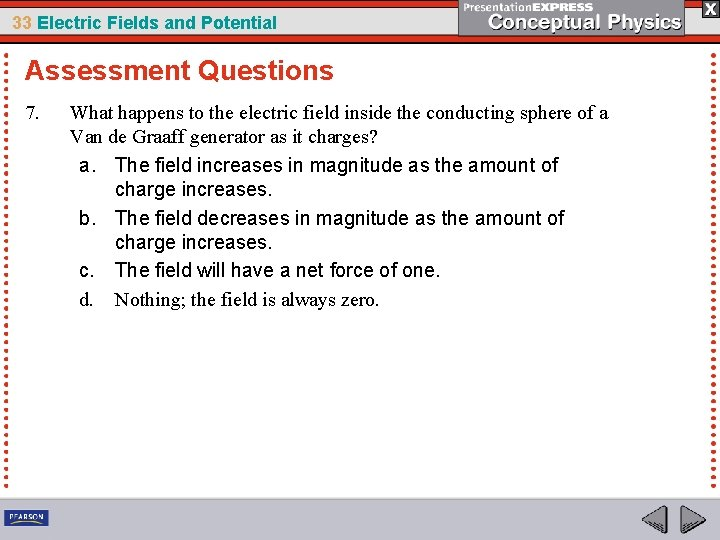33 Electric Fields and Potential Assessment Questions 7. What happens to the electric field inside the conducting sphere of a Van de Graaff generator as it charges? a. The field increases in magnitude as the amount of charge increases. b. The field decreases in magnitude as the amount of charge increases. c. The field will have a net force of one. d. Nothing; the field is always zero.33 Electric Fields and Potential Assessment Questions 7. What happens to the electric field inside the conducting sphere of a Van de Graaff generator as it charges? a. The field increases in magnitude as the amount of charge increases. b. The field decreases in magnitude as the amount of charge increases. c. The field will have a net force of one. d. Nothing; the field is always zero. Answer: D